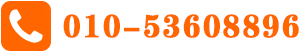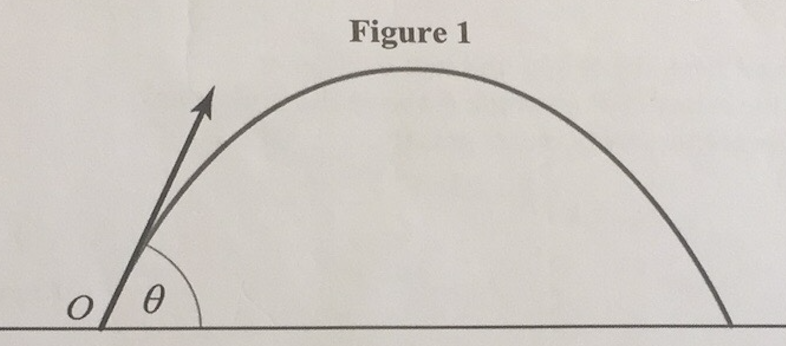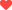# Projectile Motion典型例题解析Question： A ball is launched from the origin with speed 1m/s. Its velocity makes an angle θ above the horizontal. It travels over flat ground and is modelled as a particle moving freely under gravity. (In this question, take g=10m/s2)

A． Find the horizontal and vertical displacement of the particle at time t seconds.

B.  Show that the horizontal distant travelled by the particle before it hits the ground       is sin2θ/10.

C.  Find the value θ for which the horizontal distance travelled is a maximum.

a． 题目中给出初速度的大小和方向，因此，我们可以将其分解成竖直和水平两个方向的速度vh=1·cos θ，vv=1·sin θ，竖直方向的运动是一个加速度不变，始终向下的先减速后加速运动，水平方向是一个匀速直线运动，因此两者在运动到抛物线上任何位置，竖直和水平方向上所用的时间是相同的，根据这点是解题的关键。

b． 对于竖直方向，上升和下降两个过程可以看作往复运动，球抛出到达顶端，速度由sinθ匀减速至0，随后向下做匀加速运动，这两个过程，时间相同。运用公式可以直接解出答案。

c． 这部分运用到了数学中的计算方法，可能够证实我们在斜抛物体时，为什么呈45度的时候，抛掷的最远。

a: vh=1·cos θ，vv=1·sin θ，since 2gsh=v2-u2 ，u=sin θ-10t，

sh=cos θ·t ，sv=sin θ-t2/20

b: When the ball reaches the peak, in the vertical direction

v=0，u=sinθ, since v-u=-gt, t= sinθ/10

To the horizontal direction, when the ball hits the ground

vh=cosθ, s= vh·2t= cosθ·2· sinθ/10=2sinθ·cosθ/10=sin2θ/10

c: Since sin2θ∈[-1, 1], When sin2θ=1, the horizontal distance will be maximum,

θ=450喜欢 

## 最新文章 More## 相关推荐#### A-level基础数学知识串讲

A-level基础数学知识串讲.....

## 猜你喜欢 More